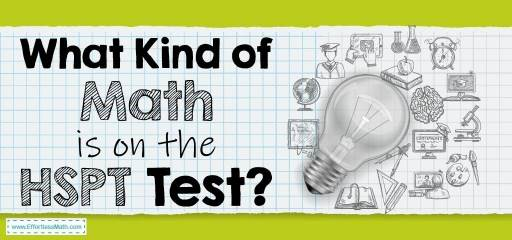# What Kind of Math Is on the HSPT Test?The HSPT (The High School Placement Test), also known as the STS-HSPT, is an admissions test designed to determine students’ ability to get admission to parochial high school. This test is formulated by the Scholastic Testing Service (STS). Participating in this test can be very important for people who are looking for admission to many private schools.

The HSPT test consists of five multiple-choice sections:

Verbal skills, quantitative skills, reading, mathematics, language.

In the HSPT test, quantitative skills and mathematics are different parts. In the quantitative skills section, test takers must answer 52 questions, including Number Series, Geometric Comparison, Non-Geometric Comparison, and Number Manipulation. In the mathematics section, students’ mathematical knowledge is assessed. The math section of the test includes arithmetic, geometry, algebra, and word problems.

## Quantitative skills

### Number Series

In this section, identifying a pattern is very important for solving sequence problems. You should be able to determine the relationship between letters, numbers, and symbols in a specific sequence. You are actually given a set of numbers and you should be able to find a number that has a significant relationship with the sequence.

### Geometric Comparison

To answer the questions in this section, you must use your geometry skills, compare geometric shapes and shaded areas, and find the best answer.

### Non-Geometric Comparison

The questions in this section involve comparing and solving multi-step equations that do not include geometry. In this section, you should read and understand the question well and then answer the questions using your math skills.

### Number Manipulation

In this area, you have to use different mathematical steps to be able to solve given problems by manipulating numbers and solutions.

## Mathematics

### Arithmetic

This section focuses on concepts such as Addition, Subtraction, Decimals, Multiplication, Division, Fractions, Order of Operations, Percentages. Understanding the basic concepts in this section is essential. The purpose of including such questions in this section is to measure your ability to perform calculations in these areas.

### Geometry

The math section of the HSPT exam does not go into the geometry section in-depth, and you will rarely encounter complex and advanced geometry questions. The geometry section tests your knowledge of topics such as Angles, Graphing, Plane Figures, Volume of Solid Figures.

### Algebra

As it turns out, in this section you are more faced with algebraic equations and how to solve problems. In algebra, you should assign variables to unknowns instead of spaces or question marks, and use algebraic rules to solve algebraic expressions.

### Word problems

In the math section of the HSPT test, you may also encounter more basic math concepts such as Word Problems: Distance, Rate, Time. Therefore, mastering these mathematical concepts is a necessity for success in this part of the HSPT Math test.

## FAQs:

### Is the HSPT Math test hard?

The HSPT test is one of the easiest tests. However, it can be very challenging if you are not prepared enough for the test.

### How do I study for the HSPT Math?

How you prepare for the HSPT Math test is very important. You can use good prep books to prepare well for the HSPT Math test. You can also use online practice tests for further practice after studying the HSPT exam courses.

### Can I bring a calculator to the HSPT test?

Some schools allow students to use basic calculators when taking the HSPT test.

### How is the HSPT Test Scored?

The HSPT scores are reported in percentiles. 76th to 99th percentiles are considered high. 24th- 75th percentiles are average, and 1st to 23rd percentiles are low. for more information, you can check here.

## More from Effortless Math for HSPT Test …

### Do you need sample practice questions to assess your math skills for the HSPT test?

Look no further! Here’s what you want: HSPT Math FREE Sample Practice Questions

### Do not know how to reduce the stress of the HSPT math test and pass the test well?

Get rid of test stress with just a few tips: Top 10 Tips to Overcome HSPT Math Anxiety

### Having trouble memorizing HSPT math test formulas?

This is a problem for many test-takers. It is best to always have a list of HSPT Math Formulas with you to better memorize them.

## Have any questions about the HSPT Test?

### What people say about "What Kind of Math Is on the HSPT Test? - Effortless Math: We Help Students Learn to LOVE Mathematics"?

No one replied yet.

X
52% OFF

Limited time only!

Save Over 52%

SAVE \$40

It was \$76.99 now it is \$36.99